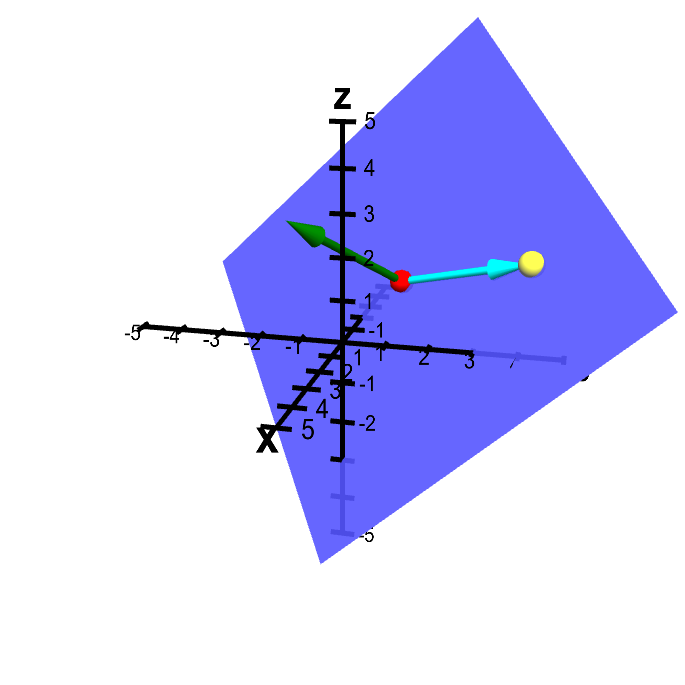# Math Insight

### Applet: Plane from point and normal vector with additional point in the planeThis plane is determined by the conditions of passing through the point $\color{red}{P}$ (in red) and being perpendicular to the vector $\color{green}{\vc{n}}$ (in green). In addition, there is another point $Q$ (in yellow) that is constrained to lie in the plane. The vector from $P$ to $Q$ is shown in cyan. You can move the points $P$ and $Q$ and the vector $\vc{n}$ by dragging them with the mouse. Dragging the mouse elsewhere rotates the whole figure. Note that the vector from $P$ to $Q$ is always perpendicular to the normal vector $\vc{n}$.